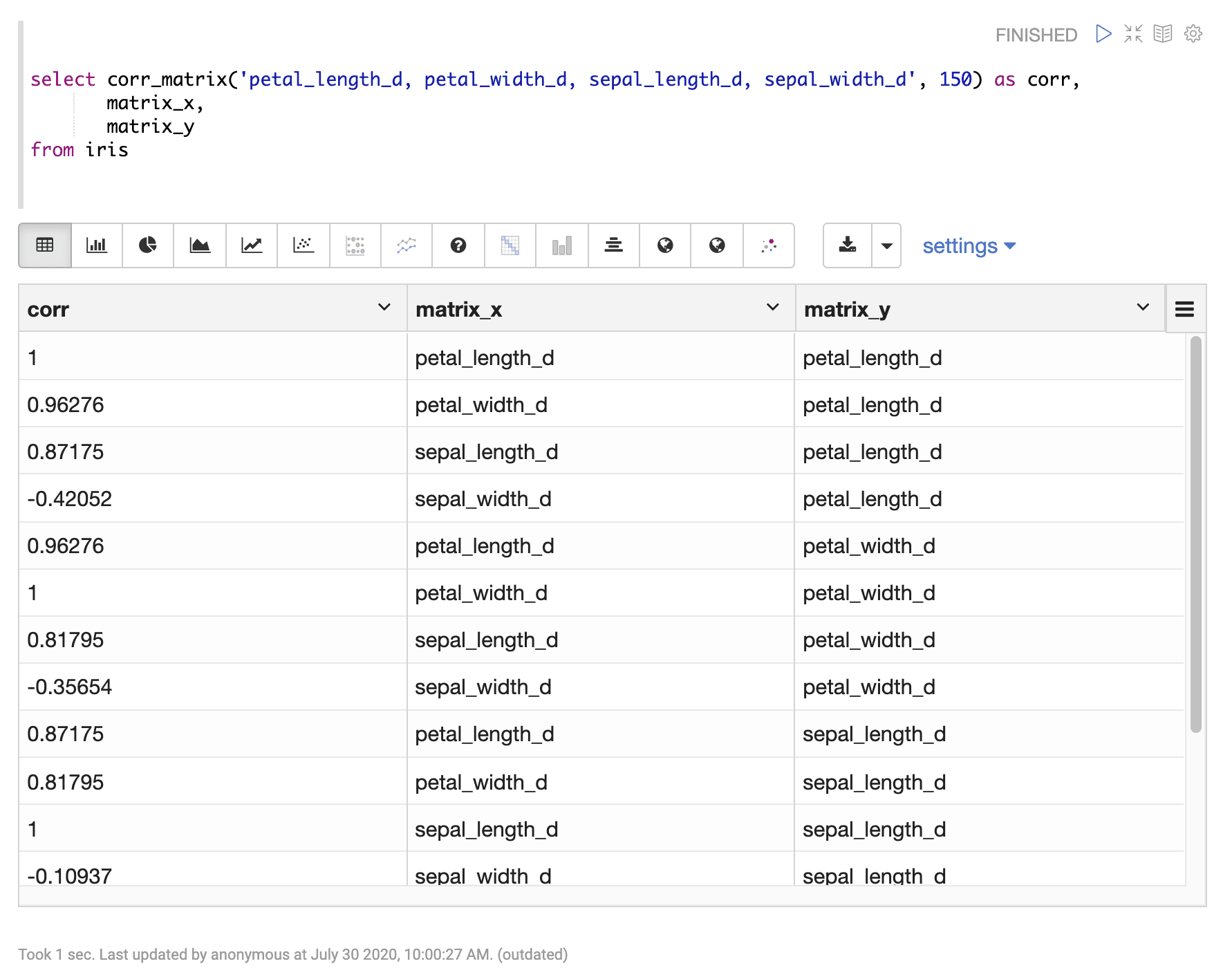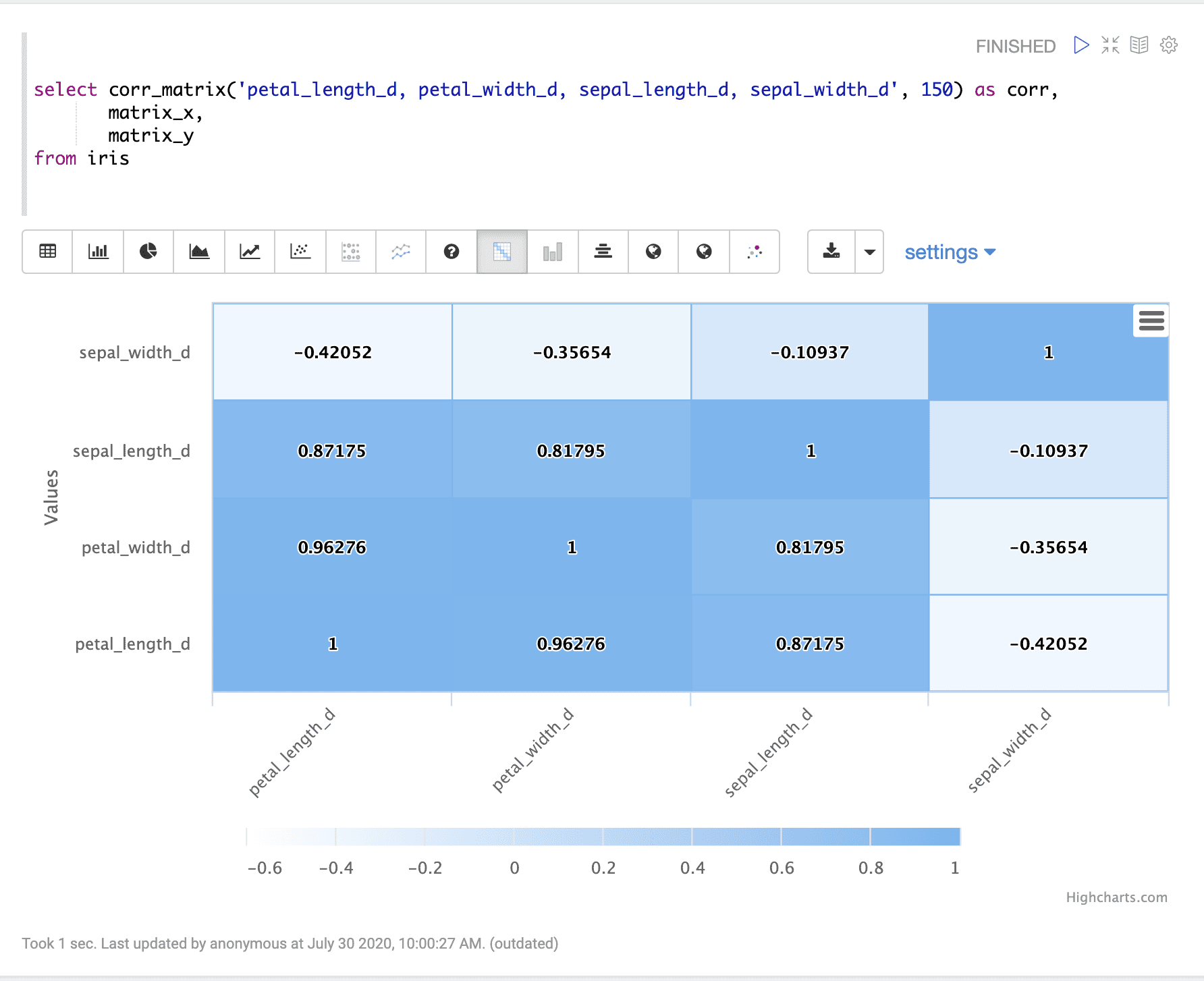# Correlation Matrices (corr_matrix)

Correlation matrices can be computed using the `corr_matrix` function. The `corr_matrix` function takes two parameters:

1. A string, enclosed in single quotes, containing a comma-separated list of numeric fields for which to calculate the matrix.

2. The sample size to compute the correlation matrix from.

## Sample syntax

``````select corr_matrix('petal_length_d, petal_width_d, sepal_length_d, sepal_width_d', 150) as corr,
matrix_x,
matrix_y
from iris``````

## Result set

The result set for the `corr_matrix` function contains one row for each two field combination listed in the first parameter. The `corr_matrix` function returns the correlation for the two field combination. There are two additional fields, `matrix_x` and `matrix_y` that contain the field combination for the row.

Sample result set in Apache Zeppelin## Visualization

The example below shows the `corr_matrix` result visualized in Apache Zeppelin with a heat map.